Question

Embedded System

On Figure P4a, you have a Common Emitter (CE) NPN BJT amplifier. Notice (unlike the circuits that we have studied in class) the absence of a source resistor R sig and load resistor R,. If we know how this L·amplifier behaves without Rsig and R, we can infer its behaviors if Rsig and R, were to be added.

You are to design the amplifier circuit on Figure P4a, i.e., find appropriate values for RB,, RB,, Rc,and R.Such that the voltage gain of the ampliifier

\left|\boldsymbol{A}_{\boldsymbol{v}}\right|=\left|\frac{\boldsymbol{v}_{\boldsymbol{p a t}}}{\boldsymbol{v}_{\boldsymbol{s} \boldsymbol{q}_{g}}}\right| \geq \mathbf{2} \mathbf{V} / \mathbf{v}

You are to design the amplifier circuit on Figure P4a, i.e., find appropriate values for RB,, RB,, Rc,and RE, such that the voltage gain of the amplifierVerified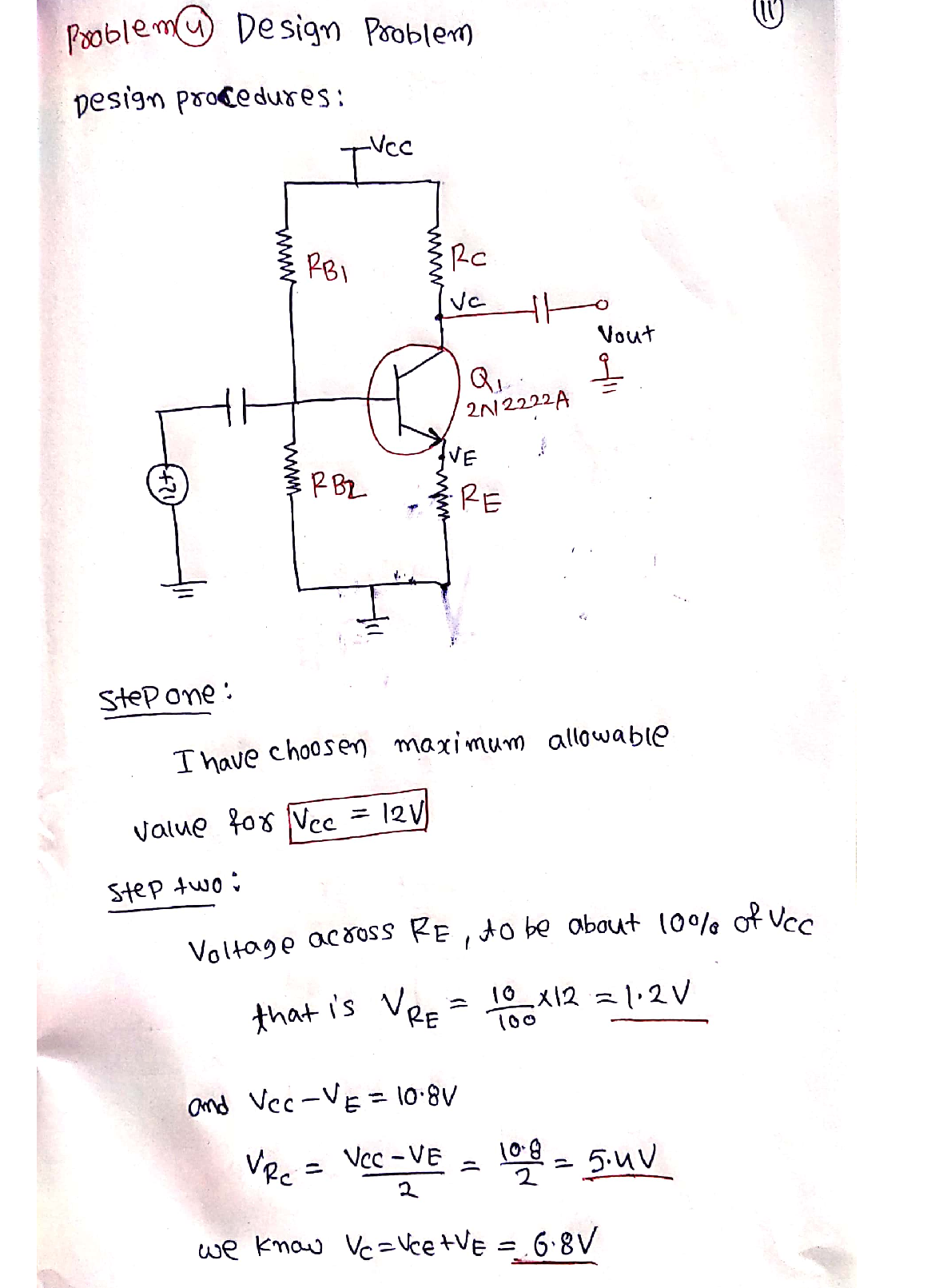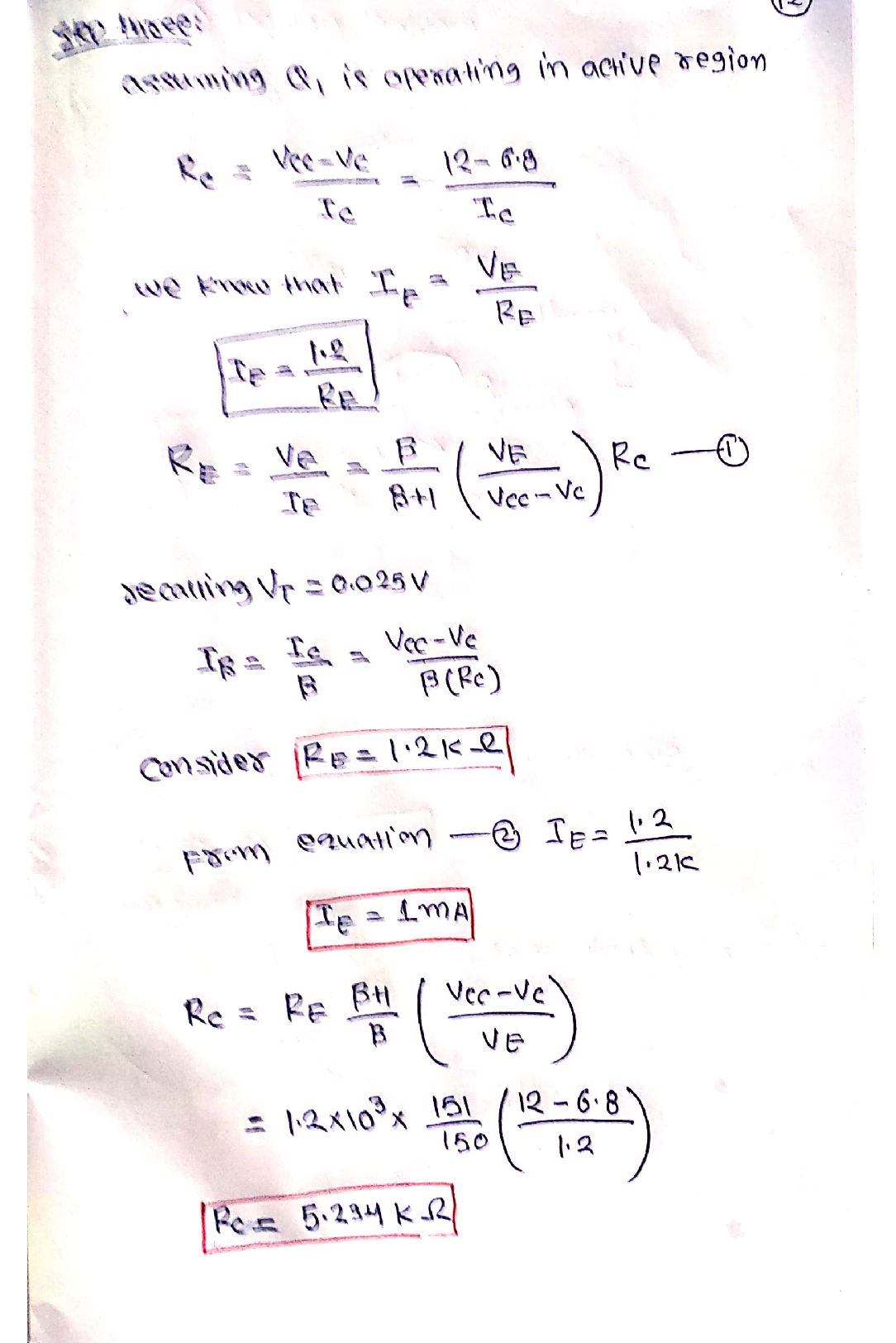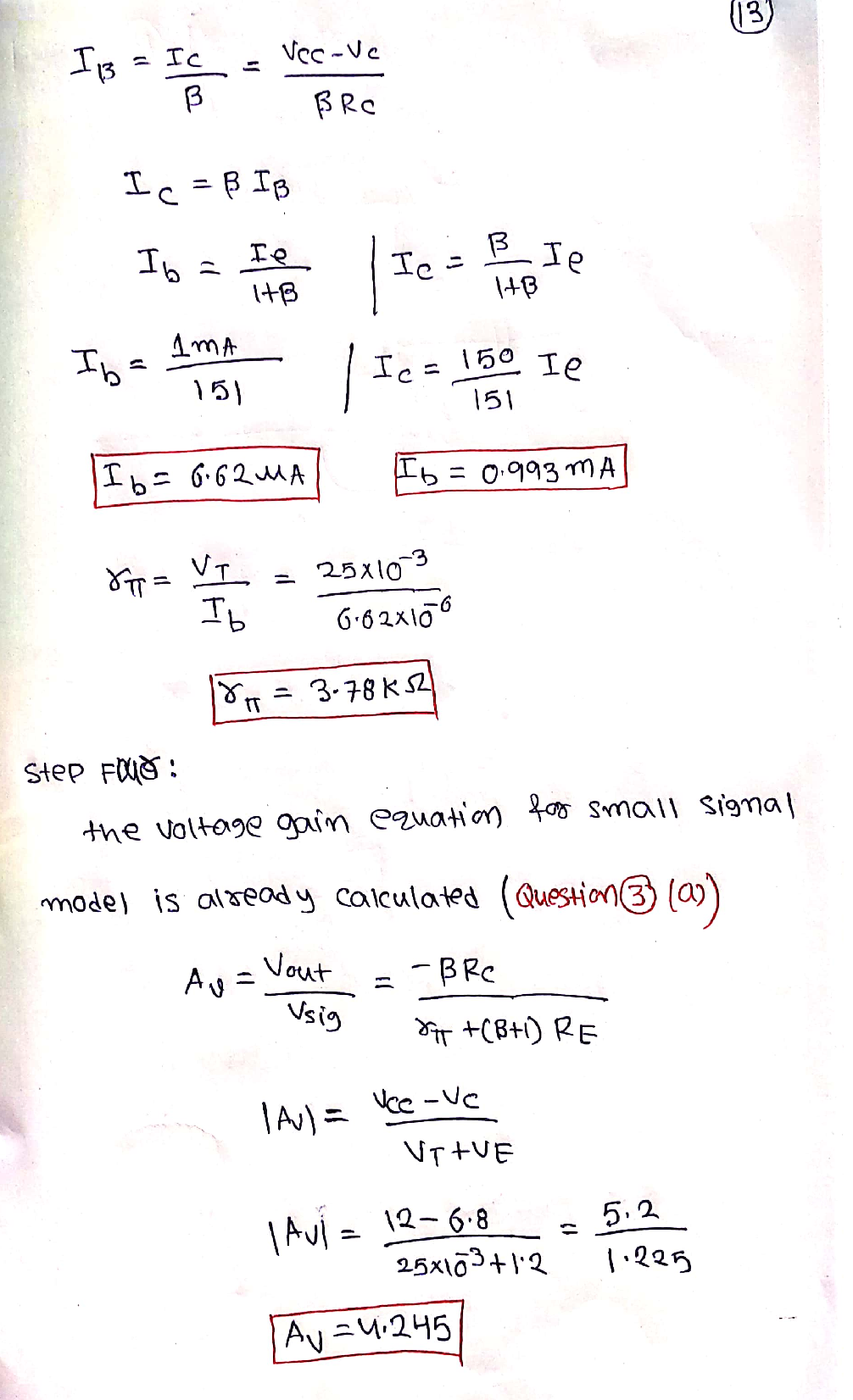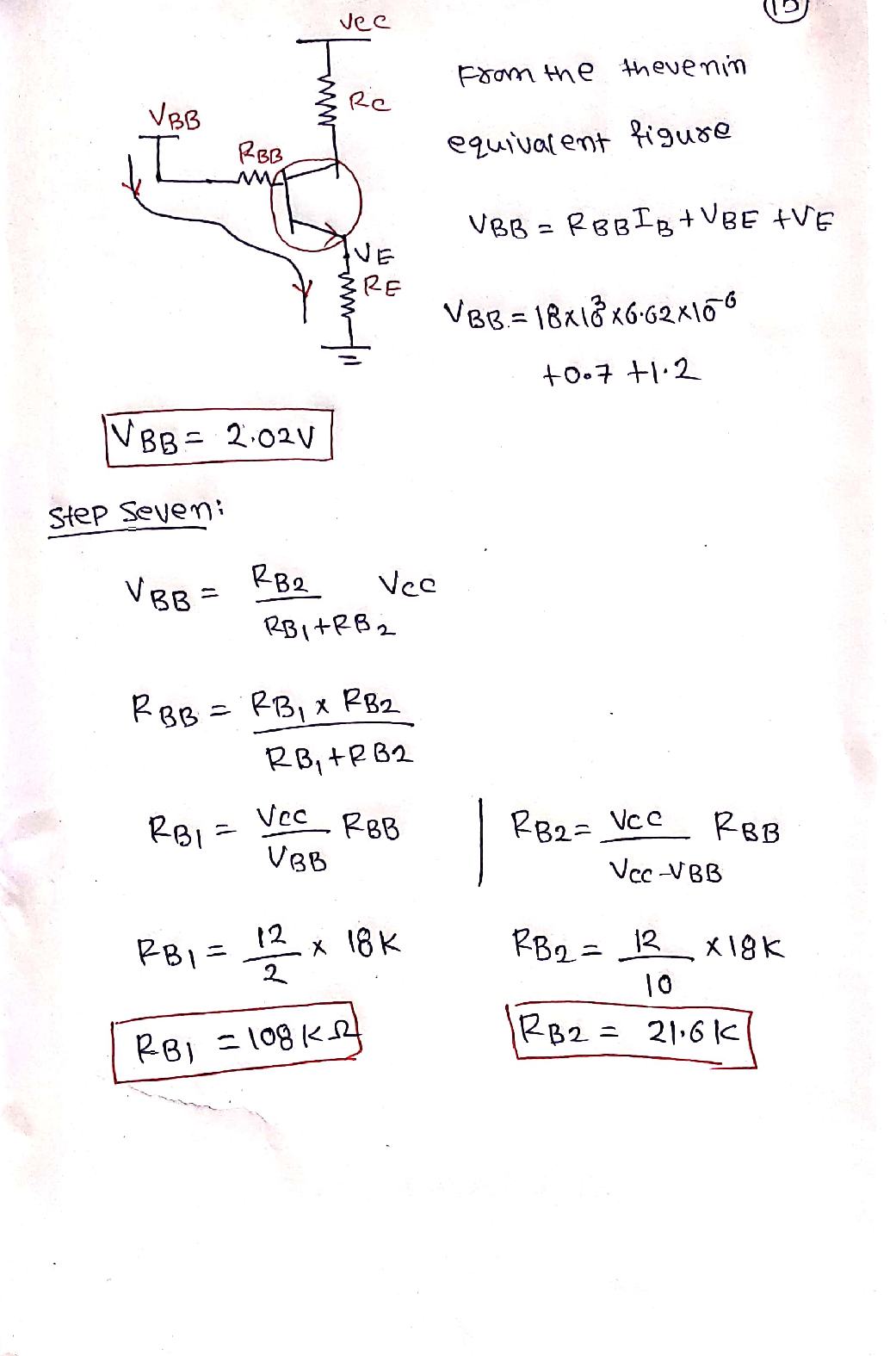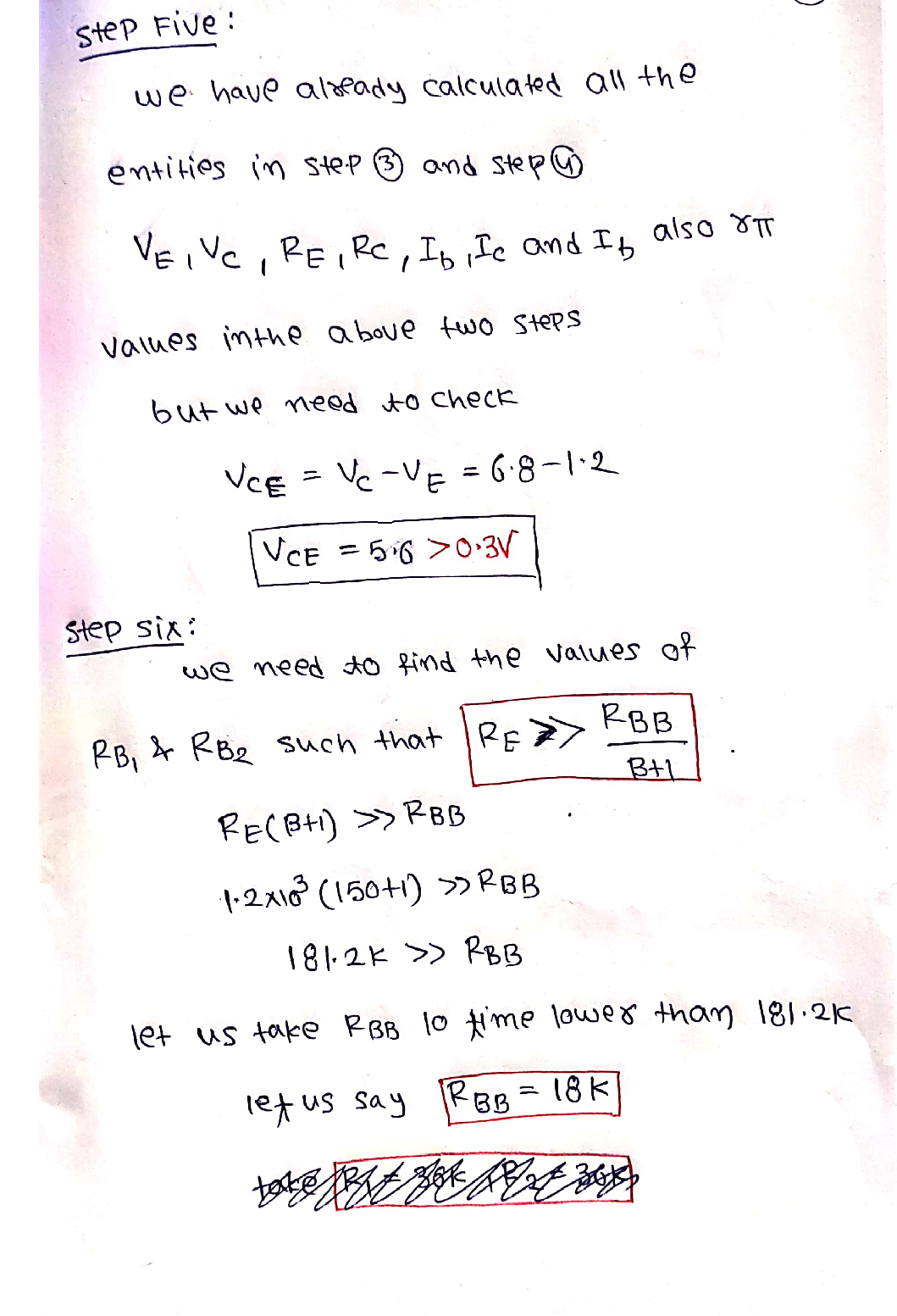### Question 26890Embedded System

A Design a 1 mH toroidal inductor on a Carbonyl W core size T30.Determine the maximum wire size that could be used to realize a single layer winding.

### Question 26889Embedded System

In ADS, select a chip inductor from the Coil Craft library with an inductance value of 80nH. Determine the maximum Q factor of the

### Question 26888Embedded System

0. Using the inductor from Problem 8, determine the self resonant frequency of the inductor and comment on the maximum frequency in which the inductor may be used in a tuned circuit application.

### Question 26887Embedded System

Design an air core inductor with an inductance value of 84 nH. Use a copper wire of 0.050 inch diameter wound on a core diameter or0.100 inch. Determine the number of turns required assuming a tight spaced winding.

### Question 26886Embedded System

Model a chip resistor (size 0603) with a resistance of 50S in ADS.Consider an application in which 502 impedance must be maintained with +10%. Create a linear analysis and determine the maximum usable frequency of the chip resistor.

### Question 26885Embedded System

) Calculate the inductance of a 5 inch length of copper flat ribbon conductor. The dimensions of the ribbon are 0.100 inches in width and 0.002 inches thick.

### Question 26884Embedded System

Calculate the reactance of the wire from Problem 2 at 10 Hz, 10MHz, and 10 GHz. Create a Linear analysis in ADS and display the wire impedance vs. frequency

### Question 26883Embedded System

Calculate the wavelength of an electromagnetic wave operating at a frequency of 428MHZ.

### Question 26685Embedded System

. The MRF911D transistor with common-emitter S-Parameters for VCE=8V and IC=10 mA
! MRF911D.S2P
! MRF911
! VCE=5V; IC=20mA
# GHz S MA R 50
! S-PARAMETER DATA
\text { So for } 0.5 \mathrm{GHz}, \mathrm{S} 11=0.54 \angle-180, \mathrm{~S} 21=6.4 \angle 85, \mathrm{~S} 12=0.06 \angle 60 \text { , and } \mathrm{S} 22=0.19 \angle-70
Design 1GHZ narrowband amplifier. Use a matching circuit with L's and C's for input andoutput matching to 50 2. (33%)

### Question 26684Embedded System

Impedance match a complex load of ZL = 25+ j15 Q to a 50 Q source impedance using a three element L-C matching pi-type network with one series inductor. Remember to perform conjugate matching for maximum power transfer. Determine the component values for operation at 300 MHz. Simulate using ADS and include results of Sl1 and S21plots up to 1 GHz. Place a marker at 500 MHz. (33 pts)

### Submit query